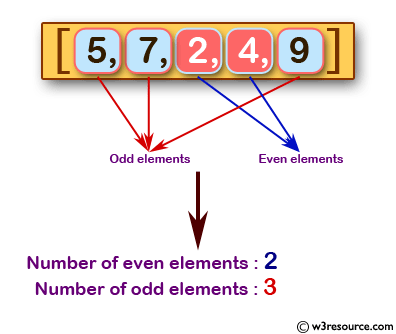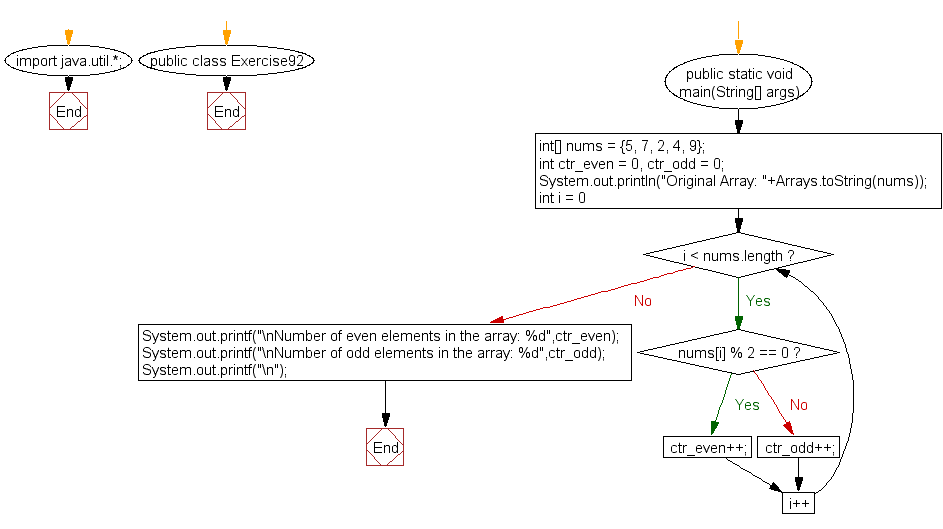﻿ Java: Count the number of even and odd elements in an array# Java Exercises: Count the number of even and odd elements in a given array of integers

## Java Basic: Exercise-92 with Solution

Write a Java program to count the number of even and odd elements in a given array of integers.

Pictorial Presentation:Sample Solution:

Java Code:

``````import java.util.*;
public class Exercise92 {
public static void main(String[] args)
{
int[] nums = {5, 7, 2, 4, 9};
int ctr_even = 0, ctr_odd = 0;
System.out.println("Original Array: "+Arrays.toString(nums));

for(int i = 0; i < nums.length; i++) {
if(nums[i] % 2 == 0)
{
ctr_even++;
}
else
ctr_odd++;
}
System.out.printf("\nNumber of even elements in the array: %d",ctr_even);
System.out.printf("\nNumber of odd elements in the array: %d",ctr_odd);
System.out.printf("\n");
}
}
```
```

Sample Output:

```Original Array: [5, 7, 2, 4, 9]

Number of even elements in the array: 2
Number of odd elements in the array: 3
```

Flowchart:Java Code Editor:

What is the difficulty level of this exercise?

Test your Programming skills with w3resource's quiz.

﻿

## Java: Tips of the Day

countOccurrences

Counts the occurrences of a value in an array.

Use Arrays.stream().filter().count() to count total number of values that equals the specified value.

```public static long countOccurrences(int[] numbers, int value) {
return Arrays.stream(numbers)
.filter(number -> number == value)
.count();
}
```

Ref: https://bit.ly/3kCAgLb